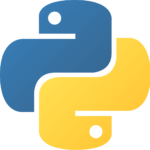# How to Convert a String to integer using PythonIn this topic, we would learn about how to convert a string to integer with and without using in-built data type. We know that a string is a collection of characters arranged sequentially enclosed within quotes and an integer is a number without any decimal points and not enclosed with any type of quotes.But when the question comes as to how to convert one data type to another, Python provides an easy way to convert into each other. In this case, we see how can we convert a string to an integer in the following order:

Using inbuilt Data Types

Suppose when I take input from the user for some reason, Python takes it and returns it as a string itself. In other words, even if someone types a number as an input, Python returns it as a string.

```name = input(" What is your name : ")
print(name)
print(type(name))

age = input("What is your age : ")
print(age)
print(type(age))```

Output:

`What is your name : Tyra`

`Tyra`

`<class 'str'>`

`What is your age : 20`

`20`

`<class 'str'>`

So you see, the type of name and age taken as the input is ‘String’.

Now, suppose if we want to add 5 to the age, we’ll do the following:

```name = input(" What is your name : ")
print(name)
print(type(name))

age = input("What is your age : ")
print(age)
print(type(age))

print(age+5)```

Output:

`What is your name : Tyra`

`Tyra`

`<class 'str'>`

`What is your age : 20`

`20`

`Traceback (most recent call last):`

`<class 'str'>`

`  File "C:/Users/prac.py", line 9, in <module>`

`    print(age+5)`

`TypeError: must be str, not int`

We can’t add 5 to age because age is of type String and we can’t do direct math with strings. So we have to change the age to an integer because we have taken age as the input and Python returns it as String.

Hence.

```name = input(" What is your name : ")
print(name)
print(type(name))

age = input("What is your age : ")
print(age)
print(type(age))

age = int(age)

print(age+5)```

Output:

`What is your name : Tyra`

`Tyra`

`<class 'str'>`

`What is your age : 20`

`20`

`<class 'str'>`

`25`

Conventional way

Suppose we don’t want to use the built-in function int() to convert string to an integer. So we have to use the conventional way to convert.

Here is a simple way to go for conversion without using int().

```"""
"123" -> 123
"-12332" -> -12332

"""

def str_to_int(input_str):

output_int = 0
if input_str == '-':
start_idx = 1
is_negative = True
else:
start_idx = 0
is_negative = False

for i in range(start_idx, len(input_str)):
place = 10**(len(input_str) - (i+1))
digit = ord(input_str[i]) - ord('0')
output_int += place * digit

if is_negative:
return -1 * output_int
else:
return output_int

s = "554"
x = str_to_int(s)
print(type(x))

s = "123"
print(str_to_int(s))

s = "-123"
print(str_to_int(s))```

Output:

`<class 'int'>`

`123`

`-123`

• First, we’ll check if the number provided by the user contains any minus sign or not, i.e. is it a negative number or not. If it contains in the first position in a minus sign, we start our conversion from the second position which contains numbers.

• Any number, let’s say 123, can be written in the form  – 10**2 * 1 + 10**1*2 + 10**0*3

• Similarly, we split each of the input number using ord(argument).

• ord(‘0’) will return 48, ord(‘1’) returns 49 etc.

• Here we use the logic that ord(‘1’) – ord(‘0) = 1, ord(‘2’) – ord(‘0’) = 2 etc. which gives us the significant number to be fetched from the given input number.

• At last, the output we get from the function is a legit integer which we converted from the given input string.

So as you can see, we can convert any string to integer either using int() function or with the conventional way.

I hope that you learned the concepts well and hence try it out to be more accurate and with this, we come to an end of this article on Converting a String to int using Python.

Got a question for us? Please mention it in the comments section of this String to Integer Tutorial and we will get back to you as soon as possible.

To get in-depth knowledge on Python along with its various applications, you can enroll for live Python online training with 24/7 support and lifetime access.

Upcoming Batches For Python Certification Training Course
Course NameDate
Python Certification Training Course

Class Starts on 28th May,2022

28th May

SAT&SUN (Weekend Batch)
View Details
Python Certification Training Course

Class Starts on 18th June,2022

18th June

SAT&SUN (Weekend Batch)
View DetailsREGISTER FOR FREE WEBINAR
Send OTPThank you for registering Join Edureka Meetup community for 100+ Free Webinars each month

### Subscribe to our Newsletter, and get personalized recommendations.#### How to Convert a String to integer using Python

edureka.co